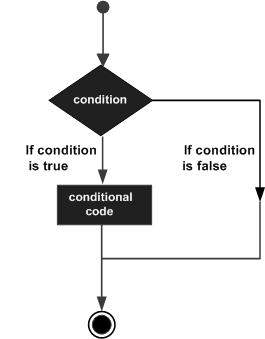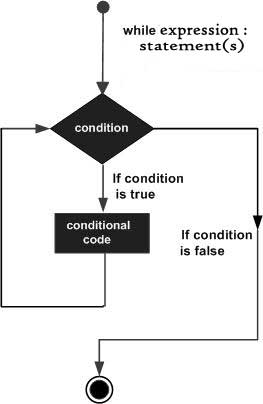# How to understand the difference between if statements and while loops

Hongtao Hao / 2020-10-13

For beginners, whichever programming language you are learning, it might be difficult to differentiate the difference between if statements and while loop. In this blog post, I’ll take Python and Javascript as examples to illustrate the differences between the two.

Both statements are made up of two parts:

1. Condition statement; and
2. Conditional code.

Conditional code will be execuated only if the condition statements are true.

Okay, those are the similaries. What about the differences? The major difference is that in an if statement, the conditional code will be execuated only once and then computers move on to the next, just like life does. However, in a while loop, after the conditional code is execuated, computers will go back to the original statement and assess it. This means that in a while loop, if the conditional code does not change validity of the condition statement, the loop will keep going forever, well, at least until your computer runs out of battery.

Let’s say we have this dataset: a = [1, 2, 3, 4, 5, 9, 10, 12, 13, 15] and we want to know how many number are there that are greater than $10$.

Let’s do it with Python first.

a = [1, 2, 3, 4, 5, 9, 10, 12, 13, 15]
num_gl_10 = 0
for n in a:
if n > 10:
num_gl_10 += 1
print(num_gl_10) #Expected output: 3


I used a for loop that iterates over the list of a. For every number in the list, the computer will first check whether it is greater than $0$. If yes, $1$ will be added to num_gl_10, which has an initial value of $0$. If not, the for loop will let the next number to be assessed (against the if condition), until all the numbers are assessed.

The logic of the if statement within the for loop is just like this:Diagram credit: Tutorialspoint

The condition here is if n > 10, and the conditoinal code here is num_gl_10 += 1.

Similarly, we can do this with Javascript:

a = [1, 2, 3, 4, 5, 9, 10, 12, 13, 15];
let num_gl_10 = 0;
for (let i = 0; i < a.length; i++) {
if (a[i] > 10) {
num_gl_10 = num_gl_10 + 1;
};
};


The logic is the same as the Python script, only that in Javascript, a here is called an array, not a list.

What if we change the if statement to be a while loop? It will be a disaster.

In Python:

a = [1, 2, 3, 4, 5, 9, 10, 12, 13, 15]
num_gl_10 = 0
for n in a:
while n > 10:
num_gl_10 += 1
print(num_gl_10)


For the first number, i.e., $1$, it will be assessed agains the condition first. Since it is smaller than 10, it won’t even get to the conditional code. Then the for loop will let the next number to be assessed. When the assessed number becomes 12, all things are changed, just like the world is during the coronavirus.

Since $12$ is greater than $10$, $1$ will be added to num_gl_10. However, different than in a if statement, our computer will assess $12$ against the condition again, and, of course, execuate the conditional code again. It will keeps doing this until our computer runs out of battery. Why? Because that’s the logic of a while loop:Diagram credit: Tutorialspoint

It will happen in Javascript as well:

a = [1, 2, 3, 4, 5, 9, 10, 12, 13, 15];
let num_gl_10 = 0;
for (let i = 0; i < a.length; i++) {
while (a[i] > 10) {
num_gl_10 = num_gl_10 + 1;
};
};


When should we use a while loop then? As I said in the beginning of this post, we use it if the conditional code will change the validity of the condition.